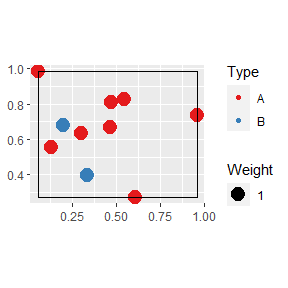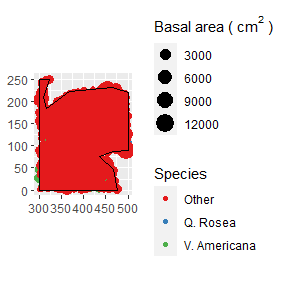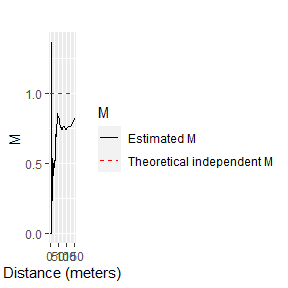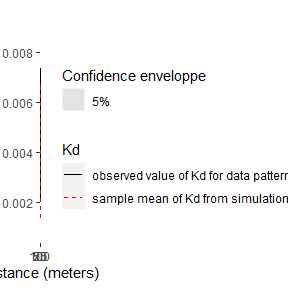# dbmss

### Distance-Based Measures of Spatial Structures

The dbmss package allows simple computation of spatial statistic functions of distance to characterize the spatial structures of mapped objects, including classical ones (Ripley’s K and others) and more recent ones used by spatial economists (Duranton and Overman’s $$K_d$$, Marcon and Puech’s $$M$$). It relies on spatstat for some core calculation.

This vignette contains a quick introduction.

# Data

The main data format is wmppp for weighted, marked point pattern. It inherits from the ppp class of the spatstat package.

A wmppp object can be created from the coordinates of points, their type and their weight.

library("dbmss")
# Draw the coordinates of 10 points
X <- runif(10)
Y <- runif(10)
# Draw the point types.
PointType   <- sample(c("A", "B"), 10, replace=TRUE)
# Plot the point pattern. Weights are set to 1 ant the window is adjusted
autoplot(wmppp(data.frame(X, Y, PointType)))An example dataset is provided: it is a point pattern from the Paracou forest in French Guiana. Two species of trees are identified, other trees are of type “Other”. Point weights are their basal area, in square centimeters.

# Plot (second column of marks is Point Types)
autoplot(paracou16,
labelSize = expression("Basal area (" ~cm^2~ ")"),
labelColor = "Species")# Main functions

The main functions of the packages are designed to calculate distance-based measures of spatial structure. Those are non-parametric statistics able to summarize and test the spatial distribution (concentration, dispersion) of points.

The classical, topographic functions such as Ripley’s K are provided by the spatstat package and supported by dbmss for convenience.

Relative functions are available in dbmss only. These are the $$M$$ and $$m$$ and $$K_d$$ functions.

The bivariate $$M$$ function can be calculated for Q. Rosea trees around V. Americana trees:

autoplot(Mhat(paracou16, , "V. Americana", "Q. Rosea"), main="")# Confidence envelopes

Confidence envelopes of various null hypotheses can be calculated. The univariate distribution of Q. Rosea is tested against the null hypothesis of random location.

autoplot(KdEnvelope(paracou16, , ReferenceType="Q. Rosea", Global=TRUE), main="")Significant concentration is detected between about 10 and 20 meters.

# Full documentation

https://ericmarcon.github.io/dbmss/